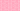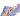PricingResources

# What is accelerated depreciation?

Written by

When an asset is new and at its most efficient, a company uses it the most. This is the guiding principle behind the accelerated depreciation method used in accounting for tax purposes. Here’s a closer look at how accelerated depreciation is calculated and when it might be beneficial.

## Accelerated depreciation definition

Accelerated depreciation is a method used to calculate asset value over time. It’s based on the principle that an asset’s value is highest at the beginning of its lifespan, allowing for more significant depreciation in value during these first few years. Several different methods are used to work out the accelerated depreciation calculation. The two main options include:

• Sum of the Years’ Digits (SYD)

• Double Declining Balance Method

We’ll explore these in greater detail below.

### Accelerated depreciation vs. straight-line depreciation

An asset’s value follows a steady trajectory over time in a straight-line depreciation method. With accelerated depreciation, the asset depreciates in cost more during the early years of its lifespan, with a slower depreciation rate later. No matter the method of depreciation, all assets should end up with the same final amount of depreciation. The main difference between accelerated depreciation vs. straight-line depreciation is timing.

## Accelerated depreciation methods

To calculate accelerated depreciation, you can use a few different methods. Here’s a little more information about the Sum of the Years’ Digits method and the Double Declining Balance method:

### Sum of the years’ digits (SYD)

The formula used in SYD calculations is as follows:

You can calculate the Sum of the Years’ Digits like so:

### Double declining balance method

With the double declining balance method, the formula is as follows:

As you can see, for the double declining balance method to work, you’ll need to know how to calculate the straight-line depreciation rate. You can use the following formula to do so:

## Are there accelerated depreciation tax benefits?

In the short term, there can be income tax benefits to using this method. While the straight-line method calculates depreciation evenly over time, businesses can deduct higher expenses during the first few years of an asset’s lifespan using the accelerated depreciation method. The expenses are then lowered as the asset is used less later in its lifespan.

For example, according to US income tax regulations, a business must use straight-line depreciation on financial statements but is able to use accelerated depreciation on income tax returns. This means that the company could deduct higher expenses on the income tax return.

At the same time, the depreciation of your company’s assets has an impact on profits. During the earlier years of an asset’s lifespan, the company would report a lower profit due to the financial loss associated with its acquisition. Then, in later years, the asset is likely to show a lower rate of depreciation, with a higher reported profit. As a result, it’s a potentially useful accounting concept for any business owner to be aware of.

## We can help

GoCardless helps you automate payment collection, cutting down on the amount of admin your team needs to deal with when chasing invoices. Find out how GoCardless can help you with ad hoc payments or recurring payments.## Interested in automating the way you get paid? GoCardless can help

Contact sales

Sales

Contact Sales

+1(415) 523-2279

Support

help@gocardless.com

+1 (628) 241-0044